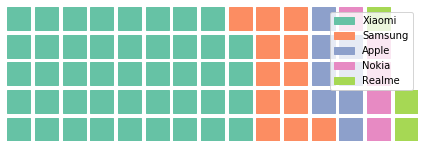Open in App
Not now

# Generate Waffle chart using pyWaffle in Python

• Last Updated : 21 Apr, 2020

A Waffle Chart is a gripping visualization technique that is normally created to display progress towards goals. Where each cell in the Waffle Chart constitutes of `10 X 10` cell grid in which each cell represents one percentage point summing up to total 100%. It is commonly an effective option when you are trying to add interesting visualization features to a visual. Waffle Charts are widely used as an Excel dashboard.

For generating Waffle Chart in Python, modules needed are – matplotlib, pandas and pyWaffle.
To install these packages, run the following commands :

```pip install matplotlib
pip install pandas
pip install pywaffle
```

Below is the implementation:

 `# python program to generate Waffle Chart ` ` `  `# importing all necessary requirements ` `import` `pandas as pd ` `import` `matplotlib.pyplot as plt ` `from` `pywaffle ``import` `Waffle ` ` `  `# creation of a dataframe ` `data ``=``{``'phone'``: [``'Xiaomi'``, ``'Samsung'``, ` `                 ``'Apple'``, ``'Nokia'``, ``'Realme'``], ` `       ``'stock'``: [``44``, ``12``, ``8``, ``5``, ``3``] ` `     ``} ` ` `  `df ``=` `pd.DataFrame(data) ` ` `  `# To plot the waffle Chart ` `fig ``=` `plt.figure( ` `    ``FigureClass ``=` `Waffle, ` `    ``rows ``=` `5``, ` `    ``values ``=` `df.stock, ` `    ``labels ``=` `list``(df.phone) ` `) `

Output:The above Waffle Chart has been generated for the data in the DataFrame